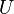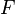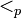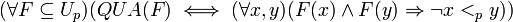# Quantization (linguistics)

In linguistics, a quantized expression is such that, whenever it is true of some entity, it is not true of any proper subparts of that entity. Example: If something is an "apple", then no proper subpart of that thing is an "apple". If something is "water", then many of its subparts will also be "water". Hence, "apple" is quantized, while "water" is not. Quantization has proven relevant to the proper characterization of grammatical telicity (roughly, sentences that present events as bounded/unbounded in time) and the mass/count distinction for nouns. The notion was first applied to linguistic semantics by the linguist Manfred Krifka.

Formally, a quantization predicate QUA can be defined as follows, where$U$ is the universe of discourse, and$F$ is a variable over sets, and$p$ is a mereological part structure on$U$ with$<_p$ the mereological part-of relation.$(\forall F\subseteq U_p)(QUA(F) \iff (\forall x,y)(F(x)\wedge F(y) \Rightarrow \neg x<_p y))$